Electron. J. Differential Equations, Vol. 2019 (2019), No. 110, pp. 1-13.

### Existence of a unique solution to an elliptic partial differential equation Diane L. Denny

Abstract:
The purpose of this article is to prove the existence of a unique classical solution to the quasilinear elliptic equation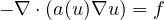for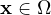, which satisfies the condition that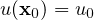at a given point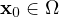, under the boundary condition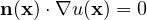for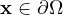where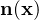is the outward unit normal vector and where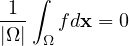. The domain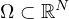is a bounded, connected, open set with a smooth boundary, and N=2 or N=3. The key to the proof lies in obtaining a priori estimates for the solution.

Submitted February 24, 2018. Published September 26, 2019.
Math Subject Classifications: 35A05
Key Words: Existence; uniqueness; quasilinear; elliptic.

Show me the PDF file (350 KB), TEX file for this article.Diane L. Denny Department of Mathematics and Statistics Texas A&M University - Corpus Christi Corpus Christi, TX 78412, USA email: diane.denny@tamucc.edu